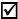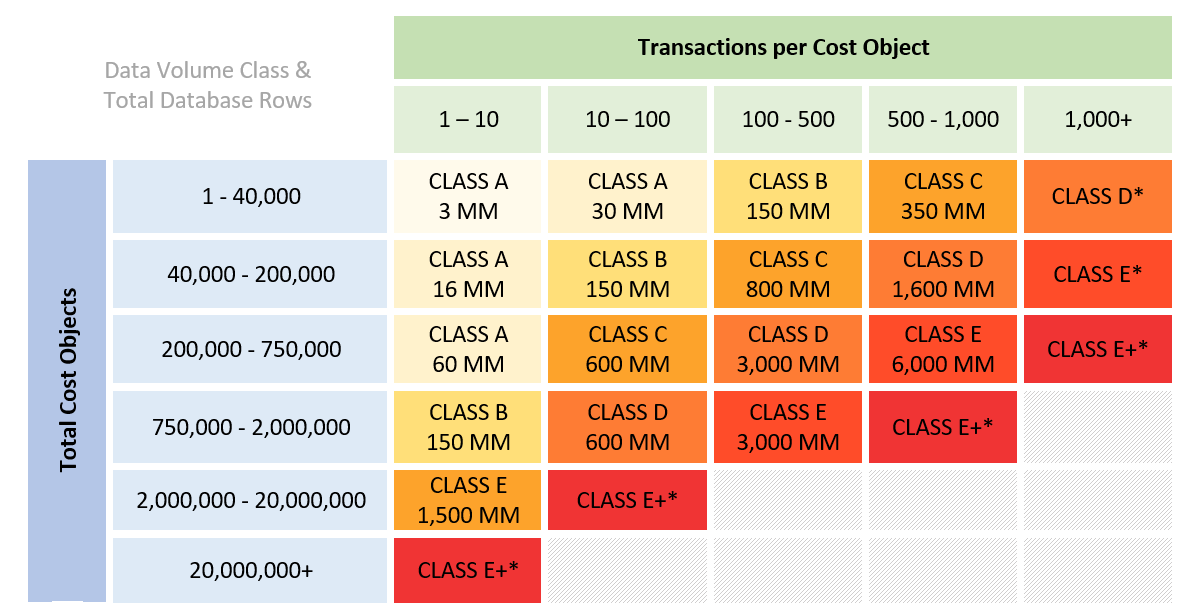# Estimating Data Volume Class - EcoSys - Help - Hexagon

## EcoSys Sizing

Language
English
Product
EcoSys
Search by Category
Help
EcoSys Version
9.2

Steps for calculating total cost objects and transactions per cost object

Follow the steps below to calculate the key measures of your anticipated data volumes.Step 1: Estimate the total number of projects (root cost objects).Step 2: Estimate the average number of WBS elements (sub cost objects) per project.Step 3: Multiple to compute Total Cost Objects (use value in the table below)Step 4: Estimate the average months (minor periods) of duration per project.Step 5: Estimate the average number of cost line items (transactions) per WBS, per month (across all versions, for example, actuals, budget, forecast, and so forth).Step 6: Multiply to compute Transaction per Cost Object (use value in the table below).

Table of Data Volume Classes

Use the graphic below to identify into which data volume class your implementation falls. This table assumes you have less than eight custom fields and/or category assignments per transaction. If you will have more than that on average, select the next highest class.